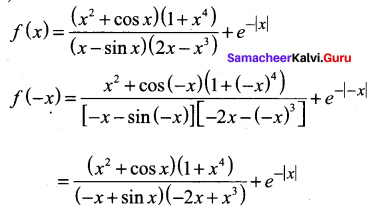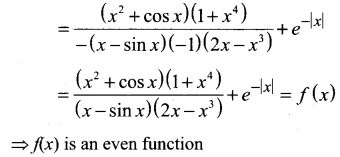# Samacheer Kalvi 11th Maths Solutions Chapter 1 Sets Ex 1.5

## Tamilnadu Samacheer Kalvi 11th Maths Solutions Chapter 1 Sets Ex 1.5

Choose the correct or the most suitable answer.

Question 1.
If A = {(x, y) : y = ex ; x ∈ R } and B = {(x, y) : y = e-x, x ∈ R } then n(A ∩ B)
(a) Infinity
(b) 0
(c) 1
(d) 2
Solution:
(c) 1
Hint.
A∩B = (0, 1)
n(A∩B) = 1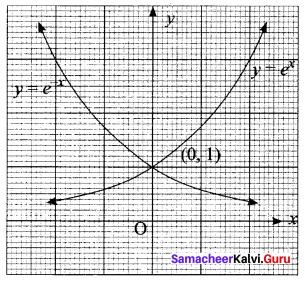Question 2.
IfA {(x, y) : y = sin x, x ∈ R) and 8= (x, y) : y = cos x, x ∈ R) then A∩B contains ……..
(a) no element
(b) infinitely many elements
(c) only one element
(d) cannot be determined.
Solution:
(b) infinitely many elementsQuestion 3.
The relation R defined on a set A = {0, -1, 1, 2} by xRy if |x2 +y2| ≤ 2, then which one of the following is true?
(a) R = {(0, 0), (0, -1), (0, 1), (-1, 0), (-1, 1), (1, 2), (1, 0)}
(b) R = {(0, 0), (0, -1), (0, 1), (-1, 0), (1, 0)
(c) Domain of R is {0, -1, 1, 2}
Solution:
(a) Range of R is {0, -1, 1}
Hint.
A= {0, -1, 1, 2}
|x2 + y2| ≤ 2
The values of x and y can be 0, -1 or 1
So range = {0, -1, 1}

Question 4.
If f(x) = |x – 2| + |x + 2|, x ∈ R, then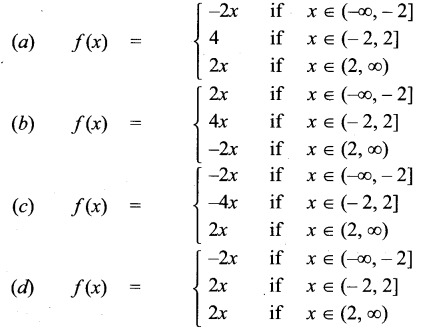Solution: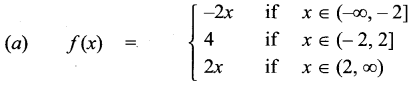Hint.
f(x) = |x – 2| + |x + 2|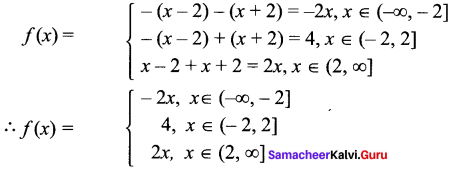Question 5.
Let R be the set of all real numbers. Consider the following subsets of the plane R x R: S = {(x, y): y = x + 1 and 0 < x < 2} and T = {(x, y) : x – y is an integer} Then which of the following is true?
(a) T is an equivalence relation but S is not an equivalence relation.
(b) Neither S nor T is an equivalence relation
(c) Both S and T are equivalence relation
(d) S is an equivalence relation but T is not an equivalence relation.
Solution:
(a) T is an equivalence relation but S is not an equivalence relation.
Hint.
(0, 1), (1, 2) it is not an equivalence relation
T is an equivalence relationQuestion 6.
Let A and B be subsets of the universal set N, the set of natural numbers. Then
A’ ∪ [(A ∩ B) ∪ B’] is ………
(a) A
(b) A’
(c) B
(d) N
Solution:
(d) N
Hint.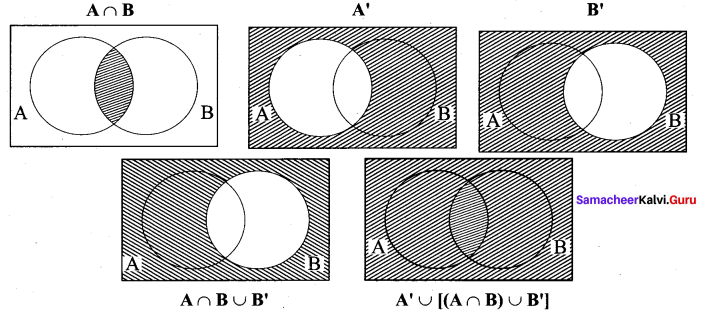Question 7.
The number of students who take both the subjects Mathematics and Chemistry is 70. This represents 10% of the enrollment in Mathematics and 14% of the enrollment in Chemistry. How many students take at least one of these two subjects?
(a) 1120
(b) 1130
(c) 1100
(d) insufficient data
Solution:
(b) 1130
Hint.
n(M ∪ C) = n(M) + n(C) – n(M ∩ C)
= 700 + 500 – 70 = 1130Question 8.
If n[(A × B) ∩ (A × C)] = 8 and n(B ∩ C) = 2 , then n(A) is
(a) 6
(b) 4
(c) 8
(d) 16
Solution:
(b) 4Question 9.
If n(A) = 2 and n(B ∪ C) = 3, then n[(A × B) ∪ (A × C)] is …….
(a) 23
(b) 32
(c) 6
(d) 5
Solution:
(c) 6
Hint.
n[(A × B) ∪ (A × C)] = n[ A × (B ∪ C)] = n(A) × n(B ∪ C) = 2 × 3 = 6

Question 10.
If two sets A and B have 17 elements in common, then the number of elements common to the set A × B and B × A is
(a) 217
(b) 172
(c) 34
(d) insufficient data
Solution:
(b) 172
Hint.
n (A ∩ B) = 17
So n [(A × B) ∩ (B × A)]
= n(A ∩ B) × n(B ∩ A) = 17 × 17 = 172Question 11.
For non-empty sets A and B, if A ⊂ B then (A × B) ∩ (B × A) is equal to ……….
(a) A ∩ B
(b) A × A
(c) B × B
(d) None of these
Solution:
(b) A × A
Hint.

When A ⊂ B, (A × B) ∩ (B × A) = A × A

Question 12.
The number of relations on a set containing 3 elements is
(a) 9
(b) 81
(c) 512
(d) 1024
Solution:
(c) 512
Hint.
Number of relations = 2n2 = 232 = 29 = 512Question 13.
Let R be the universal relation on a set X with more than one element. Then R is
(a) Not reflexive
(b) Not symmetric
(c) Transitive
(d) None of the above
Solution:
(c) Transitive

Question 14.
Let X = {1, 2, 3, 4} and R = {(1, 1), (1, 2), (1, 3), (2, 2), (3, 3), (2, 1), (3, 1), (1,4), (4, 1)}. Then R is ……..
(a) Reflexive
(b) Symmetric
(c) Transitive
(d) Equivalence
Solution:
(b) Symmetric
Hint.
(4, 4} ∉ R ⇒ R is not reflexive
(1, 4), (4, 1) ∈ R ⇒ R is symmetric
(1, 4), (4, 1) ∈ R but (4, 4) ∉ R
So R is not transitiveQuestion 15.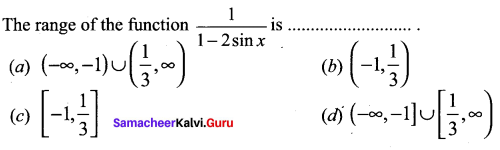Solution: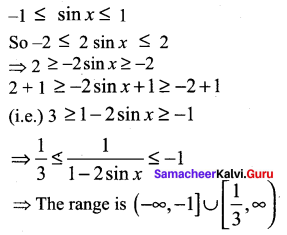Question 16.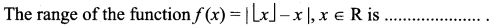Solution:
(c) [0, 1)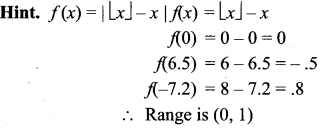Question 17.
The rule f(x) = x2 is a bijection if the domain and the co-domain are given by …..
(a) R,R
(b) R, (0, ∞)
(c) (0, ∞), R
(d) [0, ∞), [0, ∞)
Solution:
(d) [0, ∞), [0, ∞)Question 18.
The number of constant functions from a set containing m elements to a set containing n elements is
(a) mn
(b) m
(c) n
(d) m + n
Solution:
(c) n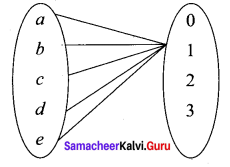Question 19.
The function f: [0, 2π] ➝ [-1, 1] defined by f(x) = sin x is
(a) One to one
(b) Onto
(c) Bijection
(d) Cannot be defined
Solution:
(b) Onto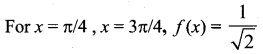So it is not one-to-one
So it is an onto functionQuestion 20.
If the function f : [-3, 3] ➝ S defined by f(x) = x2 is onto, then S is ………
(a)[-9, 9]
(b) R
(c) [-3, 3]
(d) [0, 9]
Solution:
(d) [0, 9]

Question 21.
Let X = {1, 2, 3, 4}, Y = {a, b, c, d) and f = {(1, a), (4, b), (2, c), (3, d) (2, d)}. Then f is ………
(a) An one-to-one function
(b) An onto function
(c) A function which is not one-to-one
(d) Not a function
Solution:
(d) Not a function
Hint.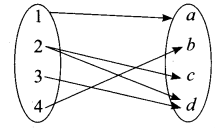Since the element 2 has two images, it is not a function

Question 22.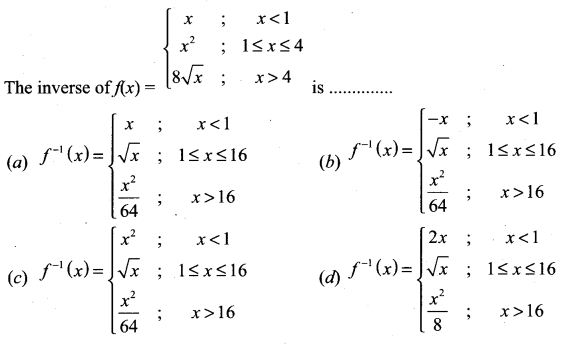Solution: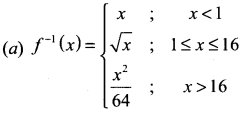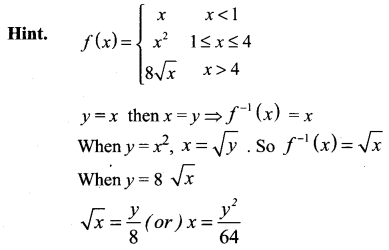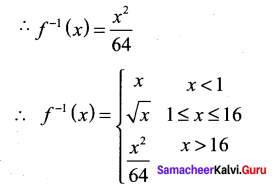Question 23.
Let f : R ➝ R be defined by f(x) = 1 – |x|. Then the range of f is ………
(a) R
(b) (1, ∞)
(c) (-1, ∞)
(d) (-∞, 1]
Solution:
(d) (-∞, 1]
Hint.
f: R ➝ R defined by
f(x) = 1 – |x|
For example,
f(1) = 1 – 1 = 0
f(8) = 1 – 8 = -1
f(-9) = 1 – 9 = -8
f(-0.2) = 1 – 0.2 = 0.8
so range = (-∞, 1]Question 24.
The function f : R ➝ R is defined by f(x) = sin x + cos x is ……
(a) An odd function
(b) Neither an odd function nor an even function
(c) An even function
(d) Both odd function and even function
Solution:
(b) Neither an odd function nor an even function

Question 25.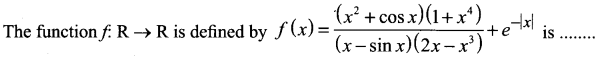(a) An odd function
(b) Neither an odd function nor an even function
(c) An even function
(d) Both odd function arid even function
Solution:
(c) An even function